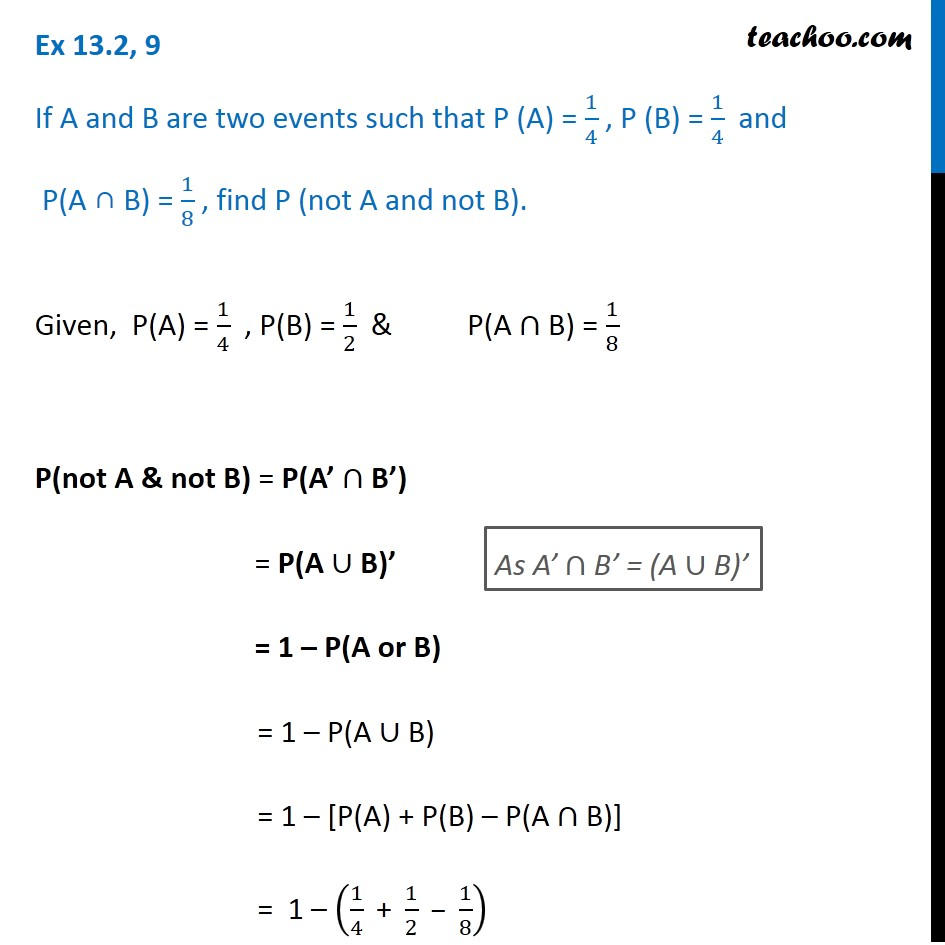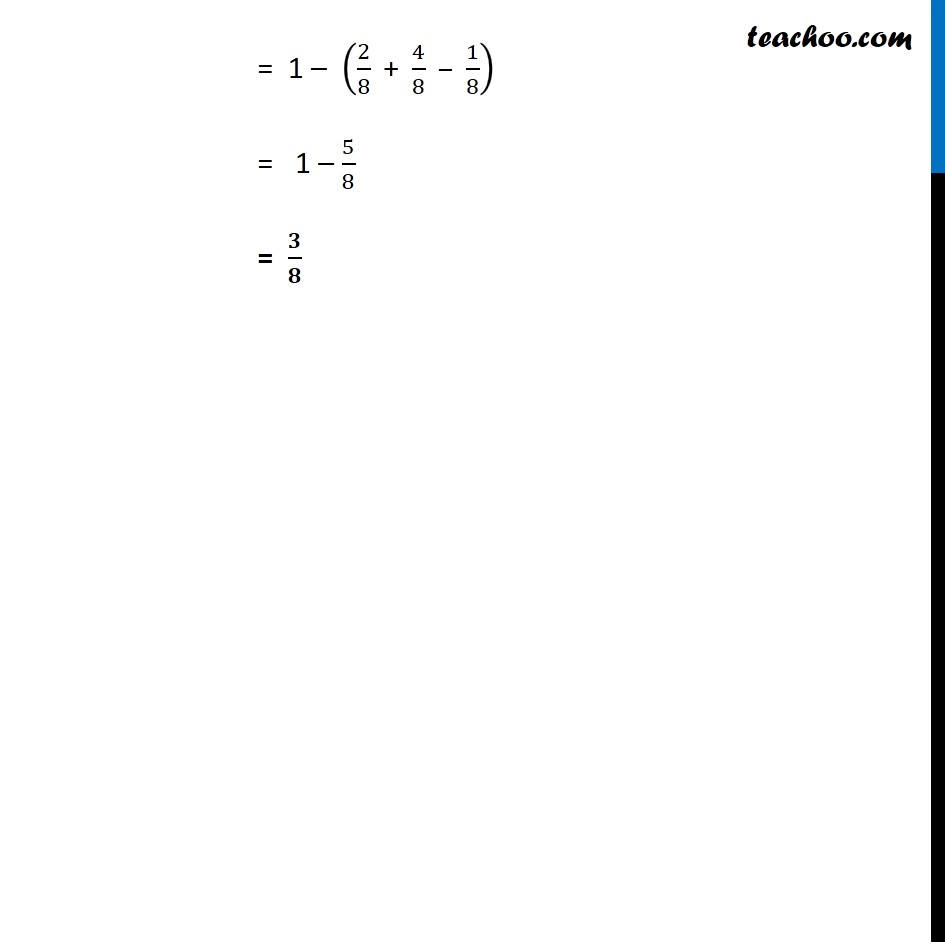Basic Probability

Chapter 13 Class 12 Probability
Concept wiseLearn in your speed, with individual attention - Teachoo Maths 1-on-1 Class

### Transcript

Ex 13.2, 9 If A and B are two events such that P (A) = 1/4 , P (B) = 1/4 and P(A ∩ B) = 1/8 , find P (not A and not B).Given, P(A) = 1/4 , P(B) = 1/2 & P(A ∩ B) = 1/8 P(not A & not B) = P(A’ ∩ B’) = P(A ∪ B)’ = 1 – P(A or B) = 1 – P(A ∪ B) = 1 – [P(A) + P(B) – P(A ∩ B)] = 1 – (1/4 " + " 1/2 – 1/8) = 1 – (2/8 " + " 4/8 – 1/8) = 1 – 5/8 = 𝟑/𝟖